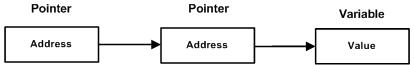### Forum

Wiki## Pascal - Pointer to Pointer

A pointer to a pointer is a form of multiple indirection, or a chain of pointers. Normally, a pointer contains the address of a variable. When we define a pointer to a pointer, the first pointer contains the address of the second pointer, which points to the location that contains the actual value as shown below.A variable that is a pointer to a pointer must be declared as such. For example,

```type
iptr =^integer;
pointerptr =^ iptr;
```

Following example would illustrate the concept as well as display the addresses:

```program exPointertoPointers;
type
iptr =^integer;
pointerptr =^ iptr;
var
num: integer;
ptr: iptr;
pptr: pointerptr;
x, y :^word;
begin
num :=3000;
(* take the address of var*)
ptr :=@num;
pptr :=@ptr;
(* let us see the value and the adresses *)
writeln('Value of num = ', num );
writeln('Value available at ptr^ = ', ptr^);
writeln('Value available at pptr^^ = ', pptr^^);
writeln('Address at ptr = ', x^);
writeln('Address at pptr = ', y^);
end.
```

When the above code is compiled and executed, it produces following result:

```Value of num = 3000
Value available at ptr^ = 3000
Value available at pptr^^ = 3000